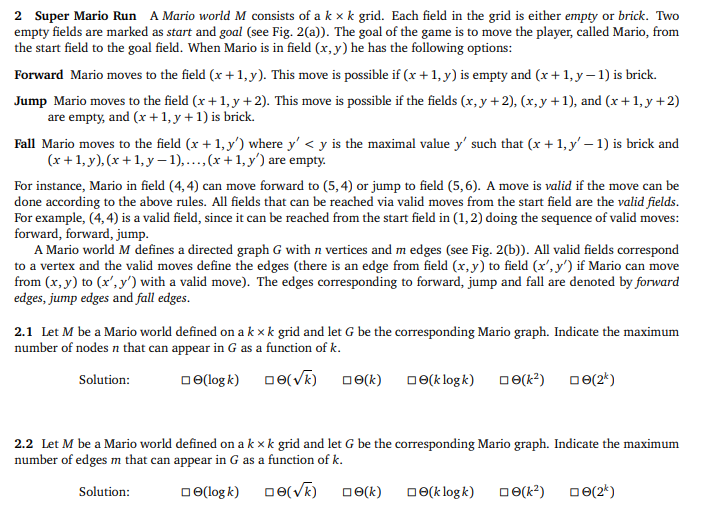# 2 Super Mario Run A Mario world M consists of a k × k grid. Each field in the grid is either empt...

Big O run time of algorithm2 Super Mario Run A Mario world M consists of a k × k grid. Each field in the grid is either empty or brick. Two empty fields are marked as start and goal (see Fig. 2(a)). The goal of the game is to move the player, called Mario, from the start field to the goal field. When Mario is in field (x, y) he has the following options Forward Mario moves to the field (x + 1,y). This move is possible if (x + 1, y) is empty and (x+1,y-1) is brick. Jump Mario moves to the field (x +1,y +2). This move is possible if the fields (x, y +2), (x,y +1), and (x +1,y +2) are empty, and (x + 1, y +1) is brick. Fall Mario moves to the field (x 1,y') where y

2.1- the answer is 6. i.e. o(2^k)

2.2- the answer is the first option i.e. o(log k)

##### Add Answer of: 2 Super Mario Run A Mario world M consists of a k × k grid. Each field in the grid is either empt...
More Homework Help Questions Additional questions in this topic.